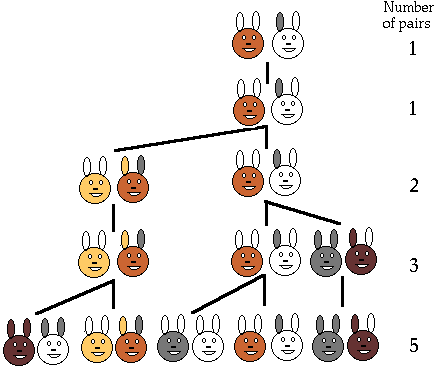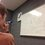# The Golden Ratio: Fibonacci NumbersHere is the previous post concerning the Golden Ratio. For a collection of all the posts concerning the Golden Ratio, click #GoldenRatio below.

Before we can discuss the Golden Ratio algebraically, we must first introduce the Fibonacci Numbers. These numbers arise from the following story: In the first year, we start with a pair of baby rabbits. It takes a year for each rabbit to grow up, so in the second year, we have a pair of adult rabbits. These rabbits then mate and have a pair of baby rabbits. For each pair of rabbits, they take a year to grow up and then mate every year after then. There is no other mating between the rabbits. This is pictured above. How many pairs of rabbits are present after $n$ years?

The way we solve this problem is by using recursion, which involves basing the next number in our sequence off of the earlier numbers. Let the first year have $f_1 = 1$ pair of rabbits. The second year has $f_2 = 1$ pairs of rabbits. And we can see that the number of pairs of rabbits in the next year will be the number of pairs of rabbits this year plus the number of pairs of rabbits that are adults this year, which is the same as the number of pairs of rabbits this year plus the number of pairs of rabbits in the prior year. (Read that slowly!) Thus we can build the recurrence relation $f_{n-2} + f_{n-1} = f_n$. Then, to answer our question, the number of pairs of rabbits that are present after $n$ years is $f_n$.

Question 1: Calculate how many pairs of rabbits there are after 11 years.

If you tried question 1, you have seen how difficult it is to calculate large Fibonacci numbers by hand. Perhaps there is a way around this...

Question 2: Write a computer program that finds the $n^{th}$ Fibonacci number. (I know that this is relatively easy, but be creative in your solution.)

However, there is an even easier method to calculating the $n^{th}$ Fibonacci number! Be before we figure that out, we need to know a bit more about this sequence's relation to the Golden Ratio. But before we do that, are there any other interesting patterns that you can find (yourself) in the Fibonacci sequence?

NextNote by Bob Krueger
7 years, 5 months ago

This discussion board is a place to discuss our Daily Challenges and the math and science related to those challenges. Explanations are more than just a solution — they should explain the steps and thinking strategies that you used to obtain the solution. Comments should further the discussion of math and science.

When posting on Brilliant:

• Use the emojis to react to an explanation, whether you're congratulating a job well done , or just really confused .
• Ask specific questions about the challenge or the steps in somebody's explanation. Well-posed questions can add a lot to the discussion, but posting "I don't understand!" doesn't help anyone.
• Try to contribute something new to the discussion, whether it is an extension, generalization or other idea related to the challenge.

MarkdownAppears as
*italics* or _italics_ italics
**bold** or __bold__ bold
- bulleted- list
• bulleted
• list
1. numbered2. list
1. numbered
2. list
Note: you must add a full line of space before and after lists for them to show up correctly
paragraph 1paragraph 2

paragraph 1

paragraph 2

[example link](https://brilliant.org)example link
> This is a quote
This is a quote
    # I indented these lines
# 4 spaces, and now they show
# up as a code block.

print "hello world"
# I indented these lines
# 4 spaces, and now they show
# up as a code block.

print "hello world"
MathAppears as
Remember to wrap math in $$ ... $$ or $ ... $ to ensure proper formatting.
2 \times 3 $2 \times 3$
2^{34} $2^{34}$
a_{i-1} $a_{i-1}$
\frac{2}{3} $\frac{2}{3}$
\sqrt{2} $\sqrt{2}$
\sum_{i=1}^3 $\sum_{i=1}^3$
\sin \theta $\sin \theta$
\boxed{123} $\boxed{123}$

Sort by:

If we create the generalized Fibonacci sequence with starting terms $a, b$ then the terms make two of their own fibonacci sequences!

$a, b, a+b, a+2b, 2a+3b, 3a+5b, 5a+8b, 8a+13b, \dots$

- 7 years, 5 months ago

Aha! After our initial discussion into Fibonacci numbers, we will be looking at the generalized Fibonacci numbers and Fibonacci-like sequences. It should be around the torque-group level.

- 7 years, 5 months ago

If you put any Fibonacci number over the one directly behind it, and use it to create a series, the values converge onto the Golden Ratio. $\frac {f(n)}{f(n - 1)} \approx \phi$. For example, $\frac {1}{1}=1$, $\frac {2}{1}=2$, $\frac {3}{2}=1.5$, $\frac {5}{3}=1.\overline 6$, $\frac {8}{5}=1.6$.

- 7 years, 5 months ago